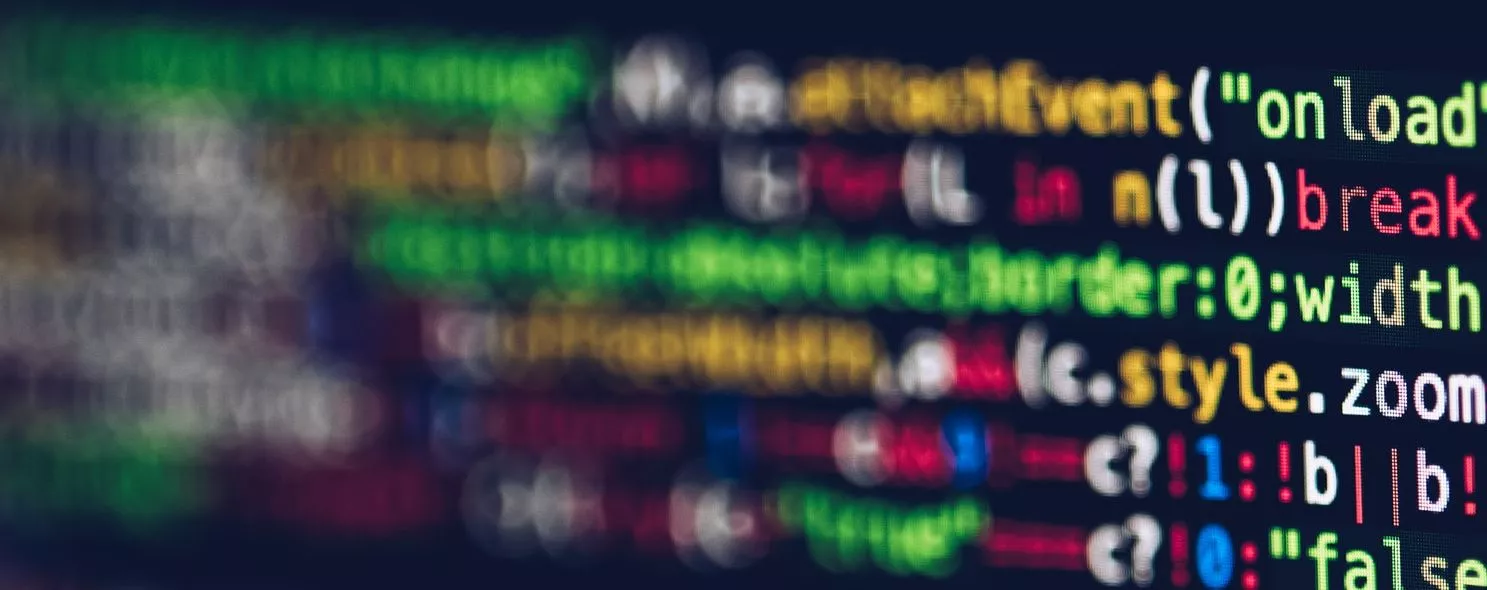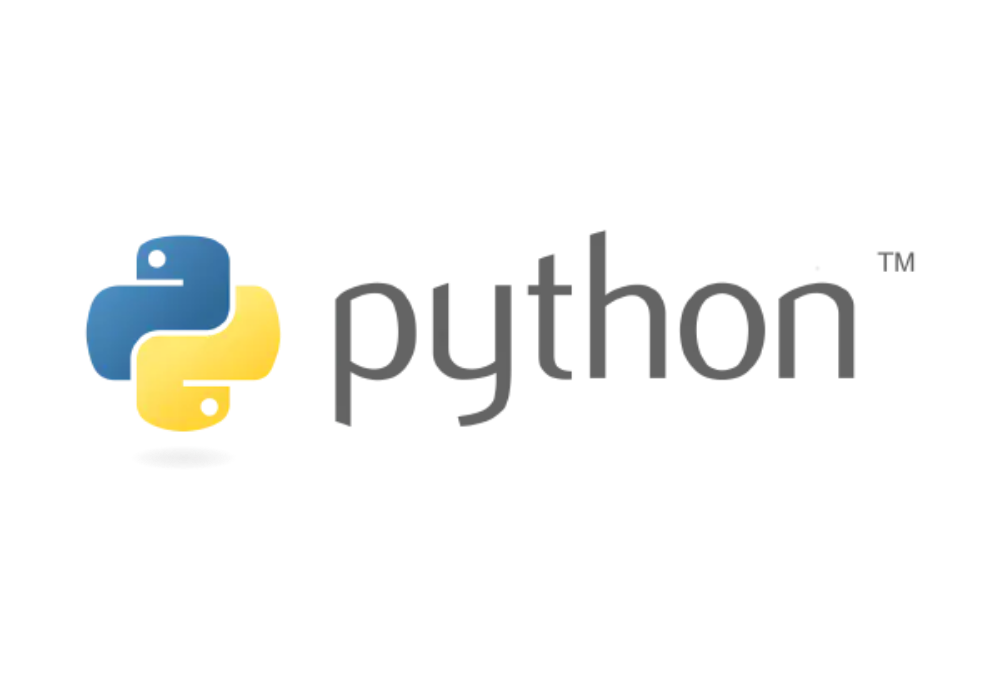Connect With Us:## There are 3 incredible return functions in PythonPython
by Admin/ on 19 Oct 2021

## There are 3 incredible return functions in Python

Today we share with you 3 relatively cold knowledge.

### The first one: the magic dictionary key

``````some_dict = {}
some_dict[5.5] = "Ruby"
some_dict[5.0] = "JavaScript"
some_dict = "Python"
``````

Output:

``````>>> some_dict[5.5]
"Ruby"
>>> some_dict[5.0]
"Python"
>>> some_dict
"Python"
``````

“Python” eliminates the existence of “JavaScript”?

💡 Description:

• The Python dictionary determines whether two keys are identical by checking for key equality and comparing hash values.

• Immutable objects with the same value always have the same hash value in Python.

Note: Objects with different values may also have the same hash (hash collision).

``````>> 5 == 5.0
True
>>> hash(5) == hash(5.0)
True
``````

When executing the statement `some_dict = "Python"`, the existing value “JavaScript” is overwritten by “Python” because Python recognizes 5 and 5.0 as the same key of `some_dict`.

### Second: return in exception handling

``````def some_func():
try:
return 'from_try'
finally:
return 'from_finally'
``````

Output:

``````>>> some_func()
'from_finally'
``````

💡 Description:

• When `return`, `break` or `continue` is executed in the try of the “try…finally” statement, the `finally` clause is still executed.

• The return value of the function is determined by the last executed `return` statement. Since the `finally` clause will always be executed, the `return` in the `finally` clause will always be the last statement executed.

### Third: Determination of identical objects

``````class WTF:
pass
``````

Output:

``````>>> WTF() == WTF() # Two different objects should not be equal
False
>>> WTF() is WTF() # also not the same
False
>>> hash(WTF()) == hash(WTF()) # The hash values should also be different
True
>>> id(WTF()) == id(WTF())
True
``````

💡 Description:

• When the `id` function is called, Python creates an object of class `WTF` and passes it to the `id` function. The id function then gets its `id` value (that is, its memory address), and discards the object. The object is then destroyed.

• When we do this twice in a row, Python allocates the same memory address to the second object. Because the `id` function (in CPython) uses the object’s memory address as the object’s id value, the id values of both objects are the same.

• In summary, an object’s id value is unique only for the life of the object. After the object is destroyed, or before it is created, other objects can have the same id value.

• So why does the `is` operation result in `False`? Let’s look at this code.

``````class WTF(object):
def __init__(self): print("I")
def __del__(self): print("D")
``````

Output:

``````>>> WTF() is WTF()
I
I
D
D
False
>>> id(WTF()) == id(WTF())
I
D
I
D
True
``````

As you can see, the order of object destruction is the reason for all the differences.